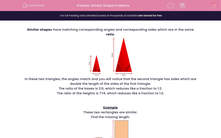# Similar Shape Problems

In this worksheet, students solve problems involving similar shapes where the scale factor is known or can be found.Key stage:  KS 2

Curriculum topic:   Maths and Numerical Reasoning

Curriculum subtopic:   2D Shapes: Triangles, Quadrilaterals and Polygons

Difficulty level:#### Worksheet Overview

Similar shapes have matching corresponding angles and corresponding sides which are in the same ratio.In these two triangles, the angles match and you will notice that the second triangle has sides which are double the length of the sides of the first triangle.

The ratio of the bases is 3:6, which reduces like a fraction to 1:2.

The ratio of the heights is 7:14, which reduces like a fraction to 1:2.

Example

These two rectangles are similar.

Find the missing length.The ratio of the bases is 4:8, which reduces to 1:2.

The big rectangle's sides are 2 times the matching sides of the small rectangle.

The missing side is 2 × 5 = 10 cm.

### What is EdPlace?

We're your National Curriculum aligned online education content provider helping each child succeed in English, maths and science from year 1 to GCSE. With an EdPlace account you’ll be able to track and measure progress, helping each child achieve their best. We build confidence and attainment by personalising each child’s learning at a level that suits them.

Get started